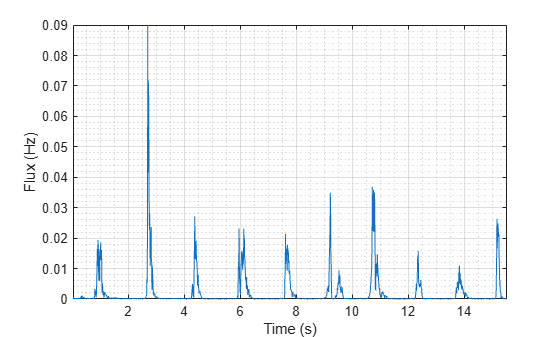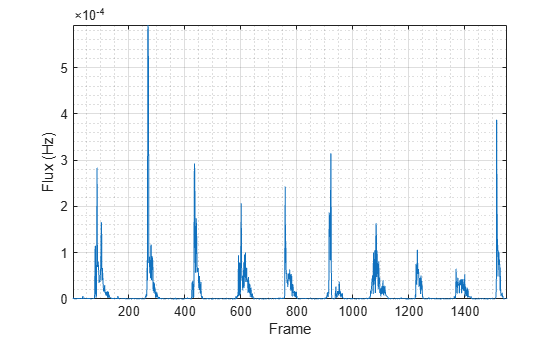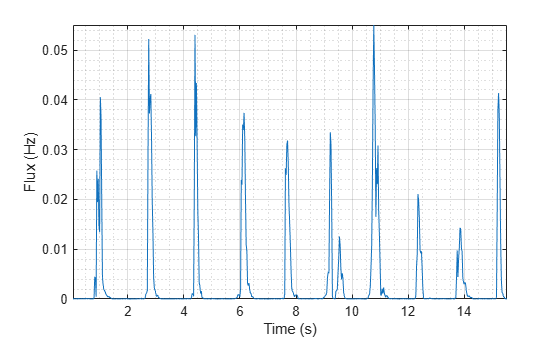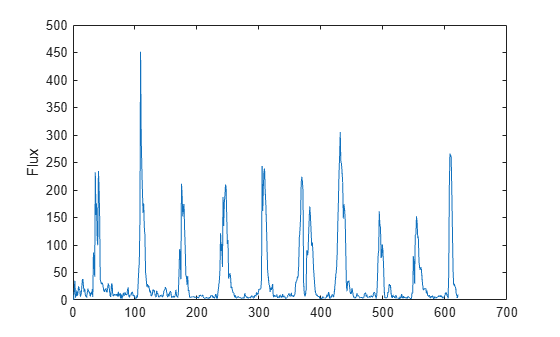# spectralFlux

Spectral flux for audio signals and auditory spectrograms

## Syntax

``flux = spectralFlux(x,f)``
``flux = spectralFlux(x,f,Name,Value)``

## Description

example

````flux = spectralFlux(x,f)` returns the spectral flux of the signal, `x`, over time. How the function interprets `x` depends on the shape of `f`.```

example

````flux = spectralFlux(x,f,Name,Value)` specifies options using one or more `Name,Value` pair arguments.```

## Examples

collapse all

Read in an audio file, calculate the flux using default parameters, and then plot the results.

```[audioIn,fs] = audioread('Counting-16-44p1-mono-15secs.wav'); flux = spectralFlux(audioIn,fs); t = linspace(0,size(audioIn,1)/fs,size(flux,1)); plot(t,flux) xlabel('Time (s)') ylabel('Flux')```Read in an audio file and then calculate the mel spectrogram using the `melSpectrogram` function. Calculate the flux of the mel spectrogram over time. Plot the results.

```[audioIn,fs] = audioread('Counting-16-44p1-mono-15secs.wav'); [s,cf,t] = melSpectrogram(audioIn,fs); flux = spectralFlux(s,cf); plot(t,flux) xlabel('Time (s)') ylabel('Flux')````[audioIn,fs] = audioread('Counting-16-44p1-mono-15secs.wav');`

Calculate the flux of the power spectrum over time. Calculate the flux for 50 ms Hamming windows of data with 25 ms overlap. Use the range from 62.5 Hz to `fs`/2 for the flux calculation. Plot the results.

```flux = spectralFlux(audioIn,fs, ... 'Window',hamming(round(0.05*fs)), ... 'OverlapLength',round(0.025*fs), ... 'Range',[62.5,fs/2]); t = linspace(0,size(audioIn,1)/fs,size(flux,1)); plot(t,flux) xlabel('Time (s)') ylabel('Flux')```Spectral flux measures the change in consecutive spectrums. To calculate spectral flux for a streaming audio signal, you must input at least two frames of audio data.

Create a `dsp.AudioFileReader` object to read in audio data frame-by-frame. Create a `dsp.AsyncBuffer` to buffer audio into overlapped frames. Create a `dsp.SignalSink` to log the spectral flux calculation.

```fileReader = dsp.AudioFileReader('Counting-16-44p1-mono-15secs.wav'); buff = dsp.AsyncBuffer; logger = dsp.SignalSink;```

In an audio stream loop:

2. Write the audio data to a `dsp.AsyncBuffer`

3. If a frame of data is available from the buffer, read a frame and one hop of data, with overlap equal to samples per frame. This represents the two most recent audio frames.

4. Calculate the spectral flux for the two most recent audio frames.

5. Log the spectral flux for later plotting. Because flux is defined by a current frame and a previous frame, and because the condition before the first frame is unknown to the function, spectral flux outputs a flux of zero for the first frame. Log only the second value output from `spectralFlux`.

```fs = fileReader.SampleRate; samplesPerFrame = round(fs*0.05); samplesOverlap = round(fs*0.025); samplesPerHop = samplesPerFrame - samplesOverlap; win = hamming(samplesPerFrame); while ~isDone(fileReader) audioIn = fileReader(); write(buff,audioIn); while buff.NumUnreadSamples >= samplesPerHop audioBuffered = read(buff,samplesPerFrame+samplesPerHop,samplesPerFrame); flux = spectralFlux(audioBuffered,fs, ... 'Window',win, ... 'OverlapLength',samplesOverlap); logger(flux(end)) end end release(fileReader)```

Plot the logged data.

```plot(logger.Buffer) ylabel('Flux')```## Input Arguments

collapse all

Input signal, specified as a vector, matrix, or 3-D array. How the function interprets `x` depends on the shape of `f`.

Data Types: `single` | `double`

Sample rate or frequency vector in Hz, specified as a scalar or vector, respectively. How the function interprets `x` depends on the shape of `f`:

• If `f` is a scalar, `x` is interpreted as a time-domain signal, and `f` is interpreted as the sample rate. In this case, `x` must be a real vector or matrix. If `x` is specified as a matrix, the columns are interpreted as individual channels.

• If `f` is a vector, `x` is interpreted as a frequency-domain signal, and `f` is interpreted as the frequencies, in Hz, corresponding to the rows of `x`. In this case, `x` must be a real L-by-M-by-N array, where L is the number of spectral values at given frequencies of `f`, M is the number of individual spectrums, and N is the number of channels.

• The number of rows of `x`, L, must be equal to the number of elements of `f`.

Data Types: `single` | `double`

### Name-Value Pair Arguments

Specify optional comma-separated pairs of `Name,Value` arguments. `Name` is the argument name and `Value` is the corresponding value. `Name` must appear inside quotes. You can specify several name and value pair arguments in any order as `Name1,Value1,...,NameN,ValueN`.

Example: `'Window',hamming(256)`

Norm type used to calculate, specified as the comma-separated pair consisting of `'NormType'` and `2` or `1`.

Data Types: `single` | `double`

### Note

The following name-value pair arguments apply if `x` is a time-domain signal. If `x` is a frequency-domain signal, name-value pair arguments are ignored.

Window applied in the time domain, specified as the comma-separated pair consisting of `'Window'` and a real vector. The number of elements in the vector must be in the range [1, `size(x,1)`]. The number of elements in the vector must also be greater than `OverlapLength`.

Data Types: `single` | `double`

Number of samples overlapped between adjacent windows, specified as the comma-separated pair consisting of `'OverlapLength'` and an integer in the range [0, `size(Window,1)`).

Data Types: `single` | `double`

Number of bins used to calculate the DFT of windowed input samples, specified as the comma-separated pair consisting of `'FFTLength'` and a positive scalar integer. If unspecified, `FFTLength` defaults to the number of elements in the `Window`.

Data Types: `single` | `double`

Frequency range in Hz, specified as the comma-separated pair consisting of `'Range'` and a two-element row vector of increasing real values in the range [0, `f`/2].

Data Types: `single` | `double`

Spectrum type, specified as the comma-separated pair consisting of `'SpectrumType'` and `'power'` or `'magnitude'`:

• `'power'` –– The spectral flux is calculated for the one-sided power spectrum.

• `'magnitude'` –– The spectral flux is calculated for the one-sided magnitude spectrum.

Data Types: `char` | `string`

## Output Arguments

collapse all

Spectral flux in Hz, returned as a scalar, vector, or matrix. Each row of `flux` corresponds to the spectral flux of a window of `x`. Each column of `flux` corresponds to an independent channel.

## Algorithms

The spectral flux is calculated as described in :

`$\text{flux(}t\text{)}={\left(\sum _{k={b}_{1}}^{{b}_{2}}{|{s}_{k}\left(t\right)-{s}_{k}\left(t-1\right)|}^{P}\right)}^{1}{P}}$`

where

• sk is the spectral value at bin k.

• b1 and b2 are the band edges, in bins, over which to calculate the spectral flux.

• P is the norm type. You can specify the norm type using `NormType`.

 Scheirer, E., and M. Slaney. "Construction and Evaluation of a Robust Multifeature Speech/Music Discriminator." IEEE International Conference on Acoustics, Speech, and Signal Processing. Volume 2, 1997, pp. 1221–1224.

﻿
##### Support